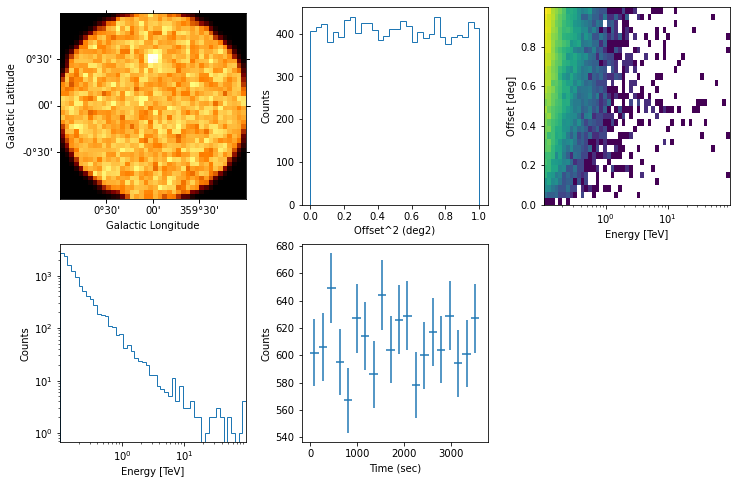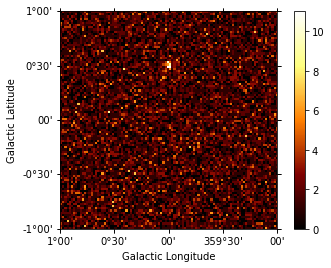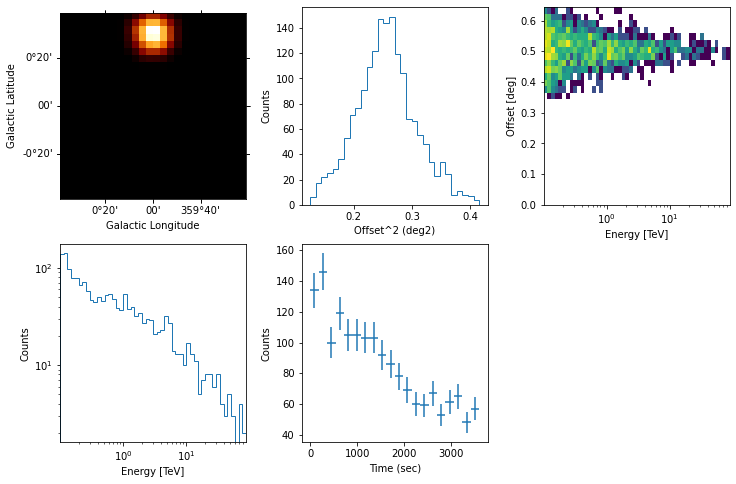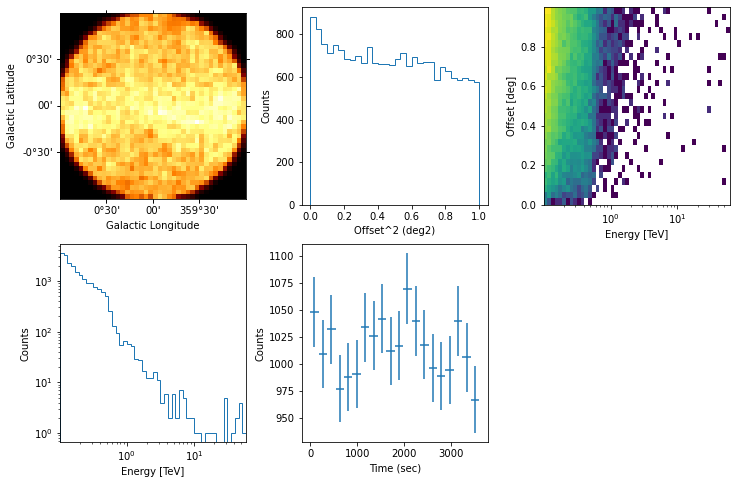This is a fixed-text formatted version of a Jupyter notebook

# Event sampling¶

## Prerequisites¶

To understand how to generate a Model and a MapDataset, and how to fit the data, please refer to the gammapy.modeling.models.SkyModel and simulate_3d.

## Context¶

This tutorial describes how to sample events from an observation of a one (or more) gamma-ray source(s). The main aim of the tutorial will be to set the minimal configuration needed to deal with the Gammapy event-sampler and how to obtain an output photon event list.

The core of the event sampling lies into the Gammapy gammapy.datasets.MapDatasetEventSampler class, which is based on the inverse cumulative distribution function (Inverse CDF).

The gammapy.datasets.MapDatasetEventSampler takes in input a gammapy.datasets.Dataset object containing the spectral, spatial and temporal properties of the source(s) of interest.

The gammapy.datasets.MapDatasetEventSampler class evaluates the map of predicted counts (npred) per bin of the given Sky model, and the npred map is then used to sample the events. In particular, the output of the event-sampler will be a set of events having information about their true coordinates, true energies and times of arrival.

To these events, IRF corrections (i.e. PSF and energy dispersion) can also further applied in order to obtain reconstructed coordinates and energies of the sampled events.

At the end of this process, you will obtain an event-list in FITS format.

## Objective¶

Describe the process of sampling events from a given Sky model and obtaining an output event-list.

## Proposed approach¶

In this section, we will show how to define an observation and to create a Dataset object. These are both necessary for the event sampling. Then, we will define the Sky model from which we sample events.

In this tutorial, we propose examples for sampling events of:

We will work with the following functions and classes:

• gammapy.data.Observations

• gammapy.datasets.Dataset

• gammapy.modeling.models.SkyModel

• gammapy.datasets.MapDatasetEventSampler

• ~gammapy.data.EventListgammapy.data.EventList

## Setup¶

:

%matplotlib inline

:

import matplotlib.pyplot as plt
from pathlib import Path
import numpy as np
import astropy.units as u
from astropy.io import fits
from astropy.table import Table
from astropy.time import Time
from astropy.coordinates import SkyCoord, Angle
from regions import CircleSkyRegion
from gammapy.data import DataStore, Observation
from gammapy.datasets import MapDataset, MapDatasetEventSampler
from gammapy.estimators import LightCurveEstimator
from gammapy.maps import MapAxis, WcsGeom, Map
from gammapy.makers import MapDatasetMaker
from gammapy.modeling import Fit
from gammapy.modeling.models import (
Model,
Models,
SkyModel,
PowerLawSpectralModel,
PowerLawNormSpectralModel,
PointSpatialModel,
TemplateSpatialModel,
ExpDecayTemporalModel,
LightCurveTemplateTemporalModel,
FoVBackgroundModel,
)


### Define an Observation¶

You can firstly create a gammapy.data.Observations object that contains the pointing position, the GTIs and the IRF you want to consider.

Hereafter, we chose the IRF of the South configuration used for the CTA DC1 and we set the pointing position of the simulated field at the Galactic Center. We also fix the exposure time to 1 hr.

:

filename = "$GAMMAPY_DATA/cta-caldb/Prod5-South-20deg-AverageAz-14MSTs37SSTs.180000s-v0.1.fits.gz" pointing = SkyCoord(0.0, 0.0, frame="galactic", unit="deg") livetime = 1 * u.hr  Now you can create the observation: :  irfs = load_cta_irfs(filename) observation = Observation.create( obs_id=1001, pointing=pointing, livetime=livetime, irfs=irfs )  ### Define the MapDataset¶ Let’s generate the gammapy.datasets.Dataset object (for more info on gammapy.datasets.Dataset objects, please visit the link): we define the energy axes (true and reconstruncted), the migration axis and the geometry of the observation. This is a crucial point for the correct configuration of the event sampler. Indeed the spatial and energetic binning should be treaten carefully and… the finer the better. For this reason, we suggest to define the energy axes (true and reconstructed) by setting a minimum binning of least 10-20 bins per decade for all the sources of interest. The spatial binning may instead be different from source to source and, at first order, it should be adopted a binning significantly smaller than the expected source size. For the examples that will be shown hereafter, we set the geometry of the dataset to a field of view of 2degx2deg and we bin the spatial map with pixels of 0.02 deg. :  energy_axis = MapAxis.from_energy_bounds( "0.1 TeV", "100 TeV", nbin=10, per_decade=True ) energy_axis_true = MapAxis.from_energy_bounds( "0.03 TeV", "300 TeV", nbin=20, per_decade=True, name="energy_true" ) migra_axis = MapAxis.from_bounds( 0.5, 2, nbin=150, node_type="edges", name="migra" ) geom = WcsGeom.create( skydir=pointing, width=(2, 2), binsz=0.02, frame="galactic", axes=[energy_axis], )  In the following, the dataset is created by selecting the effective area, background model, the PSF and the Edisp from the IRF. The dataset thus produced can be saved into a FITS file just using the write() function. We put it into the evt_sampling sub-folder: :  %%time empty = MapDataset.create( geom, energy_axis_true=energy_axis_true, migra_axis=migra_axis, name="my-dataset", ) maker = MapDatasetMaker(selection=["exposure", "background", "psf", "edisp"]) dataset = maker.run(empty, observation) Path("event_sampling").mkdir(exist_ok=True) dataset.write("./event_sampling/dataset.fits", overwrite=True)  CPU times: user 846 ms, sys: 161 ms, total: 1.01 s Wall time: 1.04 s  ### Define the Sky model: a point-like source¶ Now let’s define a Sky model (see how to create it here) for a point-like source centered 0.5 deg far from the Galactic Center and with a power-law spectrum. We then save the model into a yaml file. :  spectral_model_pwl = PowerLawSpectralModel( index=2, amplitude="1e-12 TeV-1 cm-2 s-1", reference="1 TeV" ) spatial_model_point = PointSpatialModel( lon_0="0 deg", lat_0="0.5 deg", frame="galactic" ) sky_model_pntpwl = SkyModel( spectral_model=spectral_model_pwl, spatial_model=spatial_model_point, name="point-pwl", ) bkg_model = FoVBackgroundModel(dataset_name="my-dataset") models = Models([sky_model_pntpwl, bkg_model]) file_model = "./event_sampling/point-pwl.yaml" models.write(file_model, overwrite=True)  ## Sampling the source and background events¶ Now, we can finally add the gammapy.modeling.models.SkyModel we want to event-sample to the gammapy.datasets.Dataset container: :  dataset.models = models print(dataset.models)  DatasetModels Component 0: SkyModel Name : point-pwl Datasets names : None Spectral model type : PowerLawSpectralModel Spatial model type : PointSpatialModel Temporal model type : Parameters: index : 2.000 +/- 0.00 amplitude : 1.00e-12 +/- 0.0e+00 1 / (cm2 s TeV) reference (frozen) : 1.000 TeV lon_0 : 0.000 +/- 0.00 deg lat_0 : 0.500 +/- 0.00 deg Component 1: FoVBackgroundModel Name : my-dataset-bkg Datasets names : ['my-dataset'] Spectral model type : PowerLawNormSpectralModel Parameters: norm : 1.000 +/- 0.00 tilt (frozen) : 0.000 reference (frozen) : 1.000 TeV  The next step shows how to sample the events with the gammapy.datasets.MapDatasetEventSampler class. The class requests a random number seed generator (that we set with random_state=0), the gammapy.datasets.Dataset and the gammapy.data.Observations object. From the latter, the gammapy.datasets.MapDatasetEventSampler class takes all the meta data information. :  %%time sampler = MapDatasetEventSampler(random_state=0) events = sampler.run(dataset, observation)  CPU times: user 2.15 s, sys: 453 ms, total: 2.61 s Wall time: 2.64 s  The output of the event-sampler is an event list with coordinates, energies (true and reconstructed) and time of arrivals of the source and background events. events is a ~gammapy.data.EventListgammapy.data.EventList object (more details here). Source and background events are flagged by the MC_ID identifier (where 0 is the default identifier for the background). :  print(f"Source events: {(events.table['MC_ID'] == 1).sum()}") print(f"Background events: {(events.table['MC_ID'] == 0).sum()}")  Source events: 138 Background events: 15319  We can inspect the properties of the simulated events as follows: :  events.select_offset([0, 1] * u.deg).peek()By default, the gammapy.datasets.MapDatasetEventSampler fills the metadata keyword OBJECT in the event list using the first model of the SkyModel object. You can change it with the following commands: :  events.table.meta["OBJECT"] = dataset.models.name  Let’s write the event list and its GTI extension to a FITS file. We make use of fits library in astropy: :  primary_hdu = fits.PrimaryHDU() hdu_evt = fits.BinTableHDU(events.table) hdu_gti = fits.BinTableHDU(dataset.gti.table, name="GTI") hdu_all = fits.HDUList([primary_hdu, hdu_evt, hdu_gti]) hdu_all.writeto("./event_sampling/events_0001.fits", overwrite=True)  ### Generate a skymap¶ A skymap of the simulated events can be obtained with: :  counts = Map.from_geom(geom) counts.fill_events(events) counts.sum_over_axes().plot(add_cbar=True);### Fit the simulated data¶ We can now check the sake of the event sampling by fitting the data (a tutorial of source fitting is here and here. We make use of the same gammapy.modeling.models.Models adopted for the simulation. Hence, we firstly read the gammapy.datasets.Dataset and the model file, and we fill the gammapy.datasets.Dataset with the sampled events. We set the counts map to the dataset: :  models_fit = Models.read("./event_sampling/point-pwl.yaml") dataset.counts = counts dataset.models = models_fit  Let’s fit the data and look at the results: :  %%time fit = Fit() result = fit.run(dataset) print(result)  OptimizeResult backend : minuit method : migrad success : True message : Optimization terminated successfully. nfev : 102 total stat : 76401.38 OptimizeResult backend : minuit method : migrad success : True message : Optimization terminated successfully. nfev : 102 total stat : 76401.38 CPU times: user 8.88 s, sys: 1.71 s, total: 10.6 s Wall time: 10.4 s  The results looks great! ## Time variable source using a lightcurve¶ The event sampler can also handle temporal variability of the simulated sources. In this example, we show how to sample a source characterized by an exponential decay, with decay time of 2800 seconds, during the observation. First of all, let’s create a lightcurve: :  t0 = 2800 * u.s t_ref = Time("2000-01-01T00:01:04.184") times = t_ref + livetime * np.linspace(0, 1, 100) expdecay_model = ExpDecayTemporalModel(t_ref=t_ref.mjd * u.d, t0=t0)  where we defined the time axis starting from the reference time t_ref up to the requested exposure (livetime). The bin size of the time-axis is quite arbitrary but, as above for spatial and energy binnings, the finer the better. Then, we can create the sky model. Just for the sake of the example, let’s boost the flux of the simulated source of an order of magnitude: :  spectral_model_pwl.amplitude.value = 2e-11 sky_model_pntpwl = SkyModel( spectral_model=spectral_model_pwl, spatial_model=spatial_model_point, temporal_model=expdecay_model, name="point-pwl", ) bkg_model = FoVBackgroundModel(dataset_name="my-dataset") models = Models([sky_model_pntpwl, bkg_model]) file_model = "./event_sampling/point-pwl_decay.yaml" models.write(file_model, overwrite=True)  For simplicity, we use the same dataset defined for the previous example: :  dataset.models = models print(dataset.models)  DatasetModels Component 0: SkyModel Name : point-pwl Datasets names : None Spectral model type : PowerLawSpectralModel Spatial model type : PointSpatialModel Temporal model type : ExpDecayTemporalModel Parameters: index : 2.000 +/- 0.00 amplitude : 2.00e-11 +/- 0.0e+00 1 / (cm2 s TeV) reference (frozen) : 1.000 TeV lon_0 : 0.000 +/- 0.00 deg lat_0 : 0.500 +/- 0.00 deg t0 : 2800.000 +/- 0.00 s t_ref (frozen) : 51544.001 d Component 1: FoVBackgroundModel Name : my-dataset-bkg Datasets names : ['my-dataset'] Spectral model type : PowerLawNormSpectralModel Parameters: norm : 1.000 +/- 0.00 tilt (frozen) : 0.000 reference (frozen) : 1.000 TeV  And now, let’s simulate the variable source: :  %%time sampler = MapDatasetEventSampler(random_state=0) events = sampler.run(dataset, observation)  CPU times: user 1.94 s, sys: 385 ms, total: 2.33 s Wall time: 2.33 s  :  print(f"Source events: {(events.table['MC_ID'] == 1).sum()}") print(f"Background events: {(events.table['MC_ID'] == 0).sum()}")  Source events: 1523 Background events: 15246  We can now inspect the properties of the simulated source. To do that, we adopt the select_region function that extracts only the events into a given SkyRegion of a ~gammapy.data.event_list.EventList object: :  src_position = SkyCoord(0.0, 0.5, frame="galactic", unit="deg") on_region_radius = Angle("0.15 deg") on_region = CircleSkyRegion(center=src_position, radius=on_region_radius) src_events = events.select_region(on_region)  Then we can have a quick look to the data with the peek function: :  src_events.peek()In the right figure of the bottom panel, it is shown the source lightcurve that follows a decay trend as expected. ## Extended source using a template¶ The event sampler can also work with a template model. Here we use the interstellar emission model map of the Fermi 3FHL, which can be found in the GAMMAPY data repository. We proceed following the same steps showed above and we finally have a look at the event’s properties: :  template_model = TemplateSpatialModel.read( "$GAMMAPY_DATA/fermi-3fhl-gc/gll_iem_v06_gc.fits.gz", normalize=False
)
# we make the model brighter artificially so that it becomes visible over the background
diffuse = SkyModel(
spectral_model=PowerLawNormSpectralModel(norm=5),
spatial_model=template_model,
name="template-model",
)

bkg_model = FoVBackgroundModel(dataset_name="my-dataset")

models_diffuse = Models([diffuse, bkg_model])

file_model = "./event_sampling/diffuse.yaml"
models_diffuse.write(file_model, overwrite=True)

Template file already exits, and overwrite is False

:

dataset.models = models_diffuse
print(dataset.models)

DatasetModels

Component 0: SkyModel

Name                      : template-model
Datasets names            : None
Spectral model type       : PowerLawNormSpectralModel
Spatial  model type       : TemplateSpatialModel
Temporal model type       :
Parameters:
norm                    :      5.000   +/-    0.00
tilt         (frozen)   :      0.000
reference    (frozen)   :      1.000       TeV

Component 1: FoVBackgroundModel

Name                      : my-dataset-bkg
Datasets names            : ['my-dataset']
Spectral model type       : PowerLawNormSpectralModel
Parameters:
norm                    :      1.000   +/-    0.00
tilt         (frozen)   :      0.000
reference    (frozen)   :      1.000       TeV


:

%%time
sampler = MapDatasetEventSampler(random_state=0)
events = sampler.run(dataset, observation)

CPU times: user 3.91 s, sys: 764 ms, total: 4.67 s
Wall time: 4.41 s

:

events.select_offset([0, 1] * u.deg).peek()## Simulate multiple event lists¶

In some user case, you may want to sample events from a number of observations. In this section, we show how to simulate a set of event lists. For simplicity we consider only one point-like source, observed three times for 1 hr and assuming the same pointing position.

Let’s firstly define the time start and the livetime of each observation:

:

tstarts = [1, 5, 7] * u.hr
livetimes = [1, 1, 1] * u.hr

:

%%time
for idx, tstart in enumerate(tstarts):

observation = Observation.create(
obs_id=idx,
pointing=pointing,
tstart=tstart,
livetime=livetimes[idx],
irfs=irfs,
)

dataset = maker.run(empty, observation)
dataset.models = models

sampler = MapDatasetEventSampler(random_state=idx)
events = sampler.run(dataset, observation)
events.table.write(
f"./event_sampling/events_{idx:04d}.fits", overwrite=True
)

CPU times: user 7.48 s, sys: 1.46 s, total: 8.94 s
Wall time: 8.64 s


You can now load the event list with Datastore.from_events_files() and make your own analysis following the instructions in the analysis_2 <analysis_2.ipynb>__ tutorial.

:

path = Path("./event_sampling/")
paths = list(path.rglob("events*.fits"))
data_store = DataStore.from_events_files(paths)
data_store.obs_table

:

ObservationTable length=3
ssssdegdegdegdeg
int64float64float64float64float64float64str3float64float64float64float64str13str13str13str13str1str9str13str13str31int64
225199.9999997904528800.000000209553600.03600.01.0CTA266.4049882865447-28.936177761791470.04.4527765540489235e-14NOT AVAILABLENOT AVAILABLENOT AVAILABLENOT AVAILABLEpoint-pwlNOT AVAILABLENOT AVAILABLEevent_sampling/events_0002.fits15133
03599.9999997904527200.0000002095483600.03600.01.0CTA266.4049882865447-28.936177761791470.04.4527765540489235e-14NOT AVAILABLENOT AVAILABLENOT AVAILABLENOT AVAILABLEpoint-pwlNOT AVAILABLENOT AVAILABLEevent_sampling/events_0000.fits15627
118000.0000002095521600.03600.03600.01.0CTA266.4049882865447-28.936177761791470.04.4527765540489235e-14NOT AVAILABLENOT AVAILABLENOT AVAILABLENOT AVAILABLEpoint-pwlNOT AVAILABLENOT AVAILABLEevent_sampling/events_0001.fits15226

For completeness, data_store is a gammapy.data.Datastore object. You can find more information about it here.

## Exercises¶

• Try to sample events for an extended source (e.g. a radial gaussian morphology);

• Change the spatial model and the spectrum of the simulated Sky model;

• Include a temporal model in the simulation